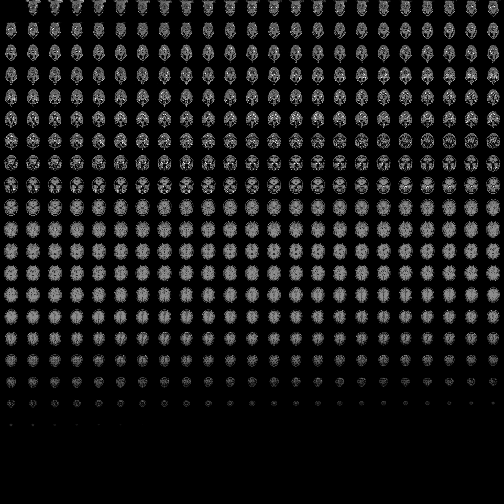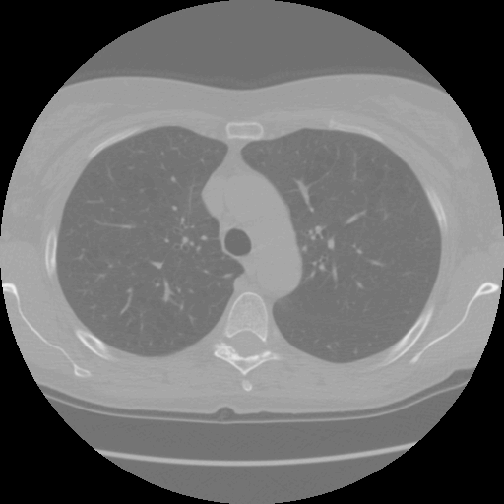Want to share your content on R-bloggers? click here if you have a blog, or here if you don't.

Lately I was getting a little bored with genomic data (and then TCGA2STAT started to give me a segfault on my university’s high performance computing facility too :stuck_out_tongue:). So I decided to analyze some brain imaging data that I had lying around instead. The first step is to do some visual data exploration. In this blog post I present some functions which I was able to find for MRI visualization in R, and which I found to be very useful. All functions presented below presuppose an image in the NIfTI data format as input, and are very user-friendly.

### Example MRI data

A T1 image obtained from http://www.jannin.org/mritemplate/ (this one to be precise) is used for example purposes in what follows. We can read it into R using the library `oro.nifti`.

```library(oro.nifti)
img <- readNIfTI("Template-T1-U8-RALPFH-BR.nii.gz", reorient = FALSE)
class(img)
##  "nifti"
## attr(,"package")
##  "oro.nifti"
dim(img)
##  182 512 512
```

### Visualize brain slices with image

The library `oro.nifti` provides a version of the generic function `image`. It allows to plot slices of the brain. The following plots the 225th axial slice of `img`.

```image(img, z = 225, plot.type = "single")
```It can also be used to draw all available slices in a single plot next to each other (but that turns out not very helpful in this case).

```image(img)
```### Display an orthographic projection with orthographic

The function `orthographic`, also from the library `oro.nifti`, gives an excellent overview of the 3D structure of the brain in two dimensions.

```orthographic(img)
```In order to indicate or emphasize a certain region of the brain, `orthographic` can be used to display a mask on top of the original image. For example, the following code chunk removes the skull from the MRI data (you need the FSL software and the fslr R package for this), and then plots the extracted brain as a mask on top of the original image.

```# skull stripping using FSL's Brain Extraction Tool (BET)
library(fslr)
img_bet <- fslbet(infile = img, retimg = TRUE)

# plotting extracted brain as a mask on top of original data
orthographic(x = img, y = mask, col.y = "lightblue")
```(Notice that the extracted brain does not fit the original image quite perfectly, but I will not pursue further improvements to the skull stripping at this point, in order to concentrate on visualization alone.)

### Polish orthographic displays with ortho2

The R package `neurobase` provides the function `ortho2` as an improved version of `orthographic`. Here we use it to overlay the brain as a semi-transparent layer on top of the original image.

```library(scales)
ortho2(img, mask, col.y = alpha("red", 0.3))
```### Show two orthographic displays side by side with double_ortho

The function `double_ortho` can be used to display two orthographic brain images of the same dimensions next to each other. As a quick demonstration we can look at the original image and the extracted brain side by side.

```brain <- img
double_ortho(img, brain)
```### Beyond MRI

Of course the same functions can be repurposed for other types of imaging data. For example I can look at CT images of the lung, where the data is taken from a current kaggle.com competition.

```library(oro.dicom)
# generate a NIfTI image from a collection of DICOMs
nii <- dicom2nifti(all_slices)
```

An `oro.nifti::image` plot of the lung:

```image(nii, z = 100, plot.type = "single")
```An `oro.nifti::orthographic` plot of the lung:

```orthographic(nii, xyz = c(200, 220, 100))
```